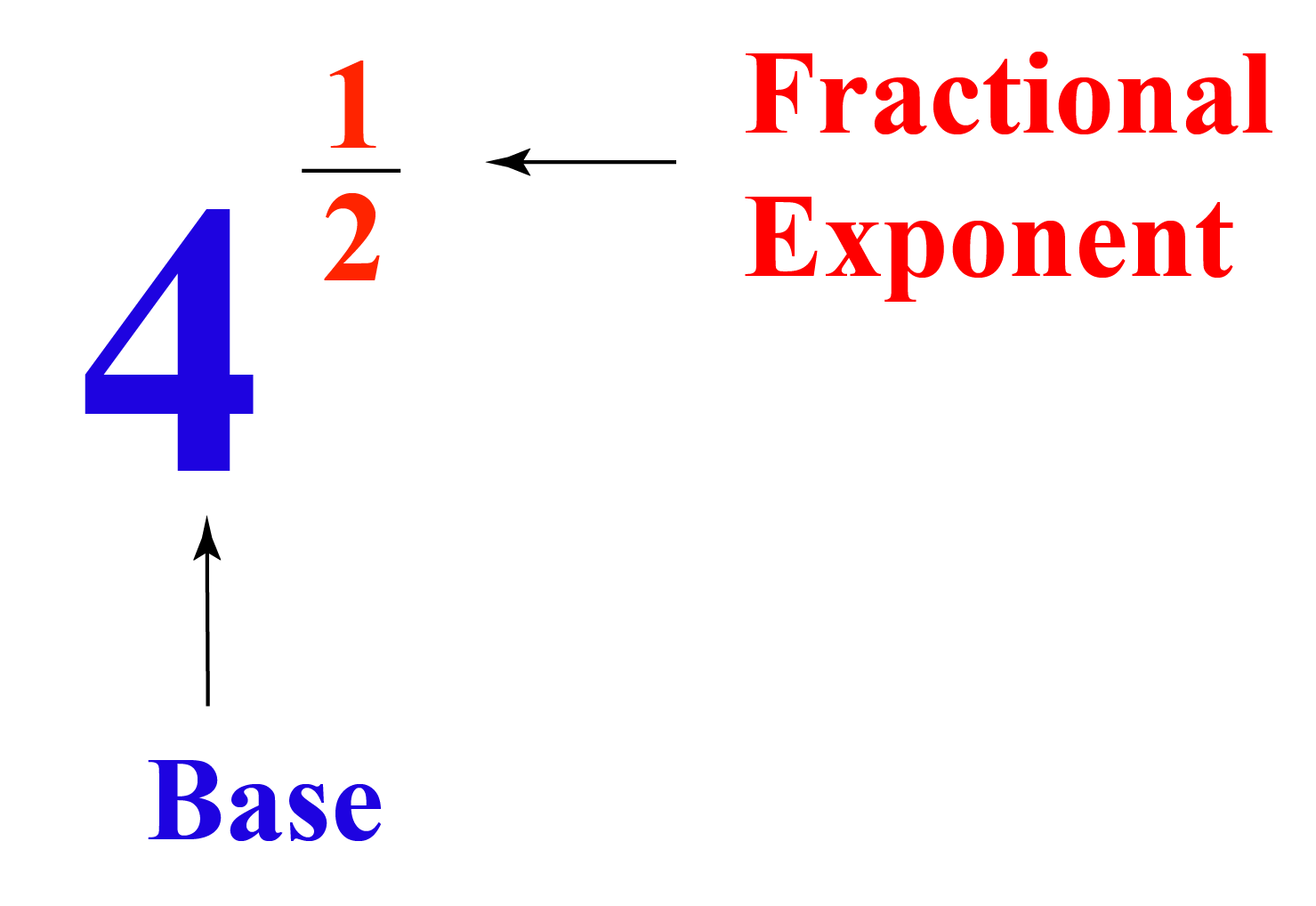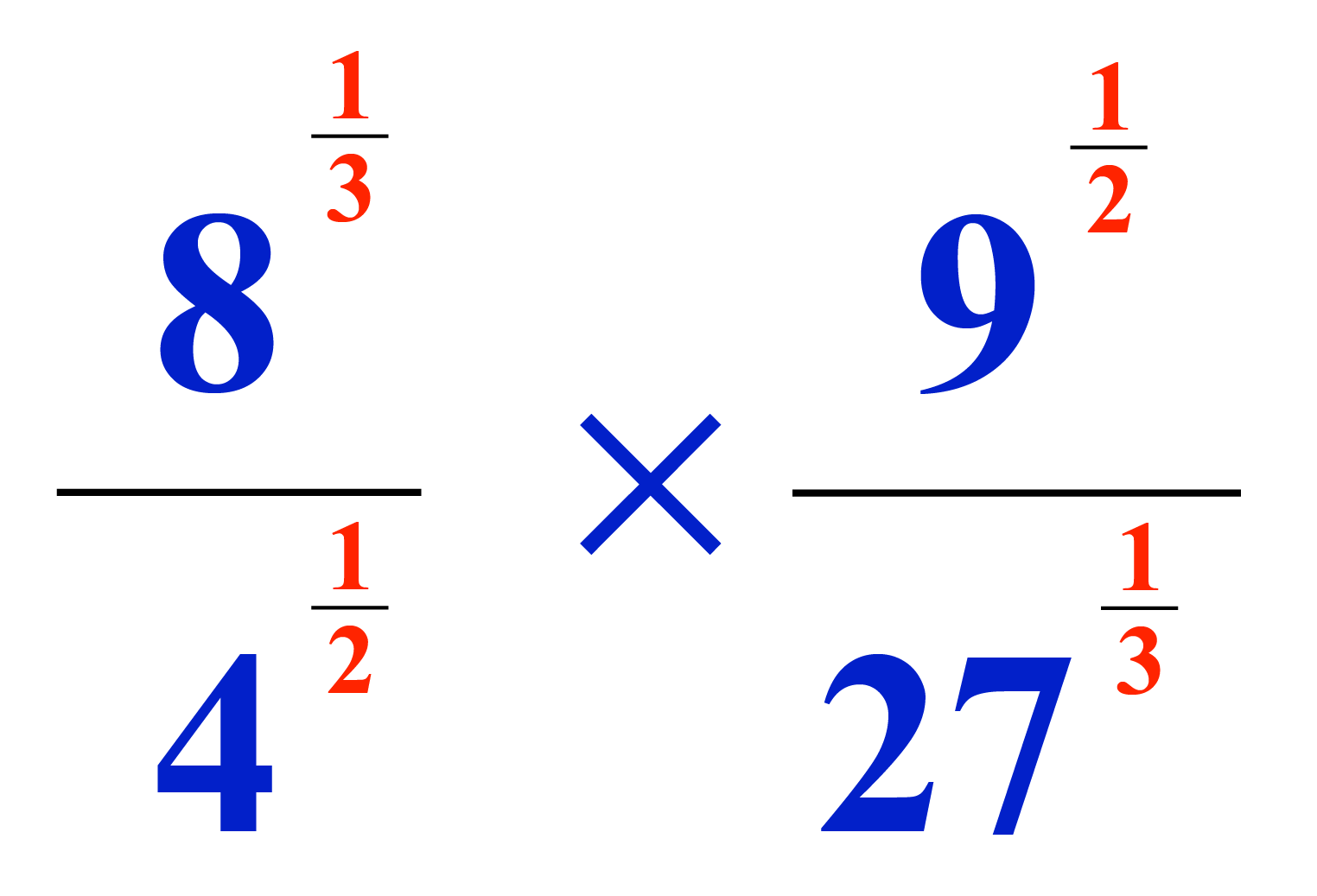# Fractional Exponents

Fractional Exponents

Before we begin this lesson on fractional exponents, we have some interesting facts about exponents for you. It is said, a form of exponential notation was used by Nicolas Chuquet in the 15th century. The term "exponent" was introduced by Michael Stifel in 1544, and Samuel Jeake then went on to introduce the term "indices" for "exponents" in 1696. This concept has been in use for years and is still considered very important today.We will begin this lesson with an overview of fractional exponents and then proceed to solving them. Let's begin!

## Lesson Plan

 1 What Are Fractional Exponents? 2 Tips and Tricks 3 Important Notes on Fractional Exponents 4 Solved Examples on Fractional Exponents 5 Interactive Questions on Fractional Exponents

## What Are Fractional Exponents?

Before we learn about fractional exponents, let's revise about exponents.

Exponents show the number of times a number is replicated in multiplication.

For example, $$4^2 = 4 \times 4 = 16$$

Here, the exponent 2 is a whole number.

If the exponent is a fraction, it is known as a fractional exponent

Look at the figure shown at the beginning of this page to understand how fractional exponents are represented.

Let's see a few examples below:

Exponent Name of the exponent Indication
$$\dfrac{1}{2}$$ Square root $$a^\frac{1}{2} = \sqrt{a}$$
$$\dfrac{1}{3}$$ Cube root $$a^\frac{1}{3} = \sqrt{a}$$
$$\dfrac{1}{4}$$ Fourth root $$a^\frac{1}{4} = \sqrt{a}$$

## How to Solve Fractional Exponents?

Let's take a look at a few examples to understand this concept.

### Examples

Example 1: Solve $$\sqrt{8} = 8^\frac{1}{3}$$

Solution: We know that 8 can be expressed as a cube of 2 which is given as, $$8 = 2^3$$.

Substituting the value of 8 in the given example we get,

$$\sqrt{2^3} = (2^3)^\frac{1}{3} = 2$$ since the product of the exponents gives $$3 \times \dfrac{1}{3} = 1$$

 $$\therefore$$ $$\sqrt{8} = 8^\frac{1}{3} = 2$$

Example 2: Solve $$\sqrt\frac{64}{125} = (\frac{64}{125})^\frac{1}{3}$$

Solution: 64 can be expressed as a cube of 4 and 125 can be expressed as a cube of 5.

They are given as, $$64 = 4^3$$ and $$125 = 5^3$$.

Substituting their values in the given example we get,

$$\sqrt\frac{4^3}{5^3} = (\frac{4^3}{5^3})^\frac{1}{3}$$

3 is a common power for both the numbers, hence $$(\frac{4^3}{5^3})^\frac{1}{3}$$ can be written as  $$((\frac{4}{5})^3)^\frac{1}{3}$$.

The equation is $$\sqrt\frac{4^3}{5^3} = ((\frac{4}{5})^3)^\frac{1}{3} = \dfrac{4}{5}$$

 $$\therefore$$ $$\sqrt\frac{64}{125} = (\frac{64}{125})^\frac{1}{3}= \dfrac{4}{5}$$

Example 3: Solve $$8^\frac{2}{3}$$

Solution: The fractional exponent shows the fraction $$\dfrac{2}{3}$$.

On simplifying the fractional exponents we get, the whole number part $$2$$ and fraction part $$\dfrac{1}{3}$$.

Hence, $$8^\frac{2}{3} = (8^2)^\frac{1}{3}$$.

64 can be expressed as a cube of 4.

Thus, $$(8^2)^\frac{1}{3} = (64)^\frac{1}{3} = (4^3)^\frac{1}{3} = 4$$

 $$\therefore$$ $$8^\frac{2}{3} = 4$$Tips and Tricks

• The $$n^{\text{th}}$$ root of any number can be expressed exponentially as $$x^\frac{1}{n} = \sqrt[n]{x}$$.
• Any negative fractional exponent $$x^{\frac{-n}{m}}$$ can be expressed as $$\dfrac{1}{x^{\fracn}{m}}}$$
• Any number $$x$$ having fractional exponent in the form of $$\dfrac{a}{b}$$, can be written as $$(x^a)^\frac{1}{b} = \sqrt[b]{x^a}$$.

## How to Multiply Fractional Exponents With Same Base?

When fractional exponents with the same base are to be multiplied, we use the Law of Exponents.

As per the Law of Product, $$x^\frac{a}{b} \times x^\frac{c}{d} = x^{\frac{a}{b}+\frac{c}{d}}$$, where $$\dfrac{a}{b}$$ and $$\dfrac{c}{b}$$ can be any fractional exponent as long as b and c are not 0.

Let's take a look at a few examples.

### Examples

Example 1: Solve $$3^\frac{1}{2} \times 3^\frac{1}{2}$$

To solve the given example, let's use the Law of Product.

Thus, $$3^\frac{1}{2} \times 3^\frac{1}{2} = 3^{\frac{1}{2}+\frac{1}{2}} = 3^1 = 3$$

 $$\therefore$$ $$3^\frac{1}{2} \times 3^\frac{1}{2} = 3$$

Example 2: Solve $$2^\frac{1}{4} \times 8^\frac{1}{4}$$

8 can be expressed as a cube of 8, i.e. 8 = 23.

$$\because$$ $$8^\frac{1}{4} = (2^3)^\frac{1}{4} = 2^\frac{3}{4}$$

Thus, $$2^\frac{1}{4} \times 2^\frac{3}{4} = 2^{\frac{1}{4}+\frac{3}{4}} = 2^1 = 2$$

 $$\therefore$$ $$2^\frac{1}{4} \times 8^\frac{1}{4} = 2$$

Example 3: Solve $$5^\frac{1}{5} \times 5^\frac{2}{5} \times 5^\frac{3}{5}$$

To solve the given example, let's use the Law of Product.

Hence, $$5^\frac{1}{5} \times 5^\frac{2}{5} \times 5^\frac{3}{5} = 5^{\frac{1}{5}+\frac{2}{5}+\frac{3}{5}} = 5^\frac{6}{5}$$

 $$\therefore$$ $$5^\frac{1}{5} \times 5^\frac{2}{5} \times 5^\frac{3}{5} = 5^\frac{6}{5}$$

## How to Divide Fractional Exponents?

Division of fractional exponents are classified into two types.

• Division of fractional exponents with different powers but the same bases
• Division of fractional exponents with the same powers but different bases

When we divide fractional exponents with different powers but same bases, we express it as $$x^\frac{a}{b} \div x^\frac{c}{d} = x^{\frac{a}{b}-\frac{c}{d}}$$.

When we divide fractional exponents with same powers but different bases, we express it as $$x^\frac{a}{b} \div y^\frac{a}{b} = (\frac{x}{y})^\frac{a}{b}$$.

Here are a few examples on division of fractional exponents.

### Examples

Example 1: Solve $$3^\frac{1}{2} \div 3^\frac{1}{4}$$

In this example, the powers are different but the bases are the same.

Hence, we can solve this problem as:

$$3^\frac{1}{2} \div 3^\frac{1}{4} = 3^{\frac{1}{2}-\frac{1}{4}} = 3^{\frac{1}{4}}$$.

 $$\therefore$$ $$3^\frac{1}{2} \div 3^\frac{1}{4} = 3^{\frac{1}{4}}$$

Example 2: Solve $$18^\frac{1}{2} \div 2^\frac{1}{2}$$

In this example, the powers are the same but the bases are different.

Hence, we can solve this problem as, $$18^\frac{1}{2} \div 2^\frac{1}{2} = (\frac{18}{2})^\frac{1}{2} = 9^{\frac{1}{2}} = \sqrt{9} = 3$$

 $$\therefore$$ $$18^\frac{1}{2} \div 2^\frac{1}{2} = 3$$

Let's have a look at the fractional exponents calculator.

## Solved Examples

 Example 1

Solve the below given question.

$$2^\frac{1}{2} \times 4^\frac{1}{4} \times 8^\frac{1}{8}$$

Solution

4 can be expressed as a square of 2, i.e. 4 = 22.

$$\because$$ $$4^\frac{1}{4} = (2^2)^\frac{1}{4} = 2^\frac{2}{4} = 2^\frac{1}{2}$$

8 can be expressed as a cube of 2, i.e. 8 = 23.

$$\because$$ $$8^\frac{1}{8} = (2^3)^\frac{1}{8} = 2^\frac{3}{8}$$

Hence, $$2^\frac{1}{2} \times 4^\frac{1}{4} \times 8^\frac{1}{8} = 2^{\frac{1}{2}+\frac{1}{2}+\frac{3}{8}} = 2^\frac{11}{8}$$

 $$\therefore$$ Answer is  $$2^\frac{11}{8}$$
 Example 2

Help Nicolas in simplifying the fractional exponents given below.Solution

Nicolas can observe that there are mainly two fractional exponents in the given question, $$\dfrac{1}{2}$$ and $$\dfrac{1}{3}$$

4 can be expressed as a square of 2, i.e. 4 = 22.

8 can be expressed as a cube of 2, i.e. 8 = 23.

9 can be expressed as a square of 3, i.e. 9 = 32.

27 can be expressed as a cube of 3, i.e. 27 = 33.

The numbers with the same powers can be written together.

Hence, the given question can be written as $$(\frac{8}{27})^\frac{1}{3} \times (\frac{9}{4})^\frac{1}{2}$$.

As per the above possible substitutions, $$((\frac{2}{3})^3)^\frac{1}{3} \times ((\frac{3}{2})^2)^\frac{1}{2}$$.

On simplifying the given fractional exponent we get, $$\dfrac{2}{3} \times \dfrac{3}{2} = 1$$

 $$\therefore$$ The answer is 1

## Interactive Questions

Here are a few activities for you to practice.

Select/Type your answer and click the "Check Answer" button to see the result.

## Let's Summarize

We hope you enjoyed learning about fractional exponents with the simulations and practice questions. Now, you will be able to easily solve problems on negative fractional exponents, adding fractional exponents, fractional exponents calculator and simplifying fractional exponents.

## About Cuemath

At Cuemath, our team of math experts are dedicated to make learning fun for our favorite readers, the students! Through an interactive and engaging learning-teaching-learning approach, the teachers explore all angles of a topic. Be it problems, online classes, videos, or any other form of relation, it’s the logical thinking and smart learning approach that we at Cuemath believe in.

## 1. How to simplify fractional exponents?

We can simplify the fractional exponents using the law of exponents.

## 2. What are negative fractional exponents?

The fractional exponents consisting of a negative sign in them are called negative fractional exponents.

More Important Topics
Numbers
Algebra
Geometry
Measurement
Money
Data
Trigonometry
Calculus